HARD
DATA STRUCTURES AND ALGORITHMS

# How to Solve Alien Dictionary

Written ByTom WagnerKenny Polyak

## Alien Dictionary Introduction

The Alien Dictionary problem involves determining whether a given order of words is valid according to an imaginary dictionary, and if so, returning a possible order for the letters used. The challenge of this problem is both in understanding how to approach the ask logically, as well as in applying graph traversal algorithms to devise a solution.

## Alien Dictionary Problem

You are given a list of lexicographically sorted words from an alien language. This language, despite sharing a character set with ASCII, has a unique order. Return the alphabetical order of all the letters found in the list of words. There may be more than one possible answer.

### Example Inputs and Outputs

Output: "kdac"

#### Example 2

Input: words = ["b","a"]
Output: "ba"

#### Example 3

Input: words = ["ab","a","b"]
Output: ""

### Constraints

• 0 <= words.length <= 100
• 0 <= words[i].length <= 100
• The character set for `words[i]` is ASCII.

## Alien Dictionary Solutions

Some graph problems look like graph problems: the problem domain is classicaly graph-related (networking, travel between cities, etc), the task is to explicitly build a graph, or you're given a graph data structure as an input. The crux of this problem, however, is to see a graph where there isn't an obvious graph.

And it really isn't obvious - a list of strings does not immediately scream graph. How can we approach a problem like this in an interview setting and discover the graph? First, start by carefully understanding what the problem offers us. The given words are lexicographically ordered, which implies that a relative relationship between some the characters exists. We are asked to find a possible order for the characters in the list of words, and since there could be more than one order, this alerts us that this could be a kind of pathfinding problem - the imagined path being a valid order of all the characters following their corresponding relationships in the list of words.

Graphs come in many forms, so let's think concretely about what our graph might look like in this case. A graph is simply a collection of nodes (vectors), but what makes it come to life are the connections (edges) between the vectors: each vector contains a reference to any other vectors it may be connected to in the graph. Importantly, each vector is not organized absolutely within the graph - unlike, say, an array, where each member is indexed globally - but connections can exist between any two vectors, indicating either a bi-directional relationship (like a friend) or a directional relationship (like a one-way street). Since our input is ordered, it makes sense to represent the characters using a directed graph. Rather than trying to decipher exactly where a given character falls in the global order, we can try to figure out what character it comes before or after based on the relative lexicographical order.

Before moving on, we should also clarify with our interviewer if the input will always be "valid" - or in other words, if it will always result in a valid output. There are a couple edges cases we might want to defend against if the input is not always friendly. First, the problem asks us to return an alphabetical order for all the characters included in the input. We can imagine a case where a character within our graph is unreachable - due to a cycle - so this would indicate a character whose alphabetical order remains inconclusive. Second, it seems possible that the input can contain words followed by their own prefix, like in the case of "ab" and "a". This, too, will be invalid, since in a valid alphabet, prefixes would have precedence.

Let's divide our work into three steps: 1) Derive relational character data from the input list; 2) Build a graph based on these relations; 3) Traverse the graph to solve the problem (we'll consider two different approaches for this step).

Step 1: Derive relationships between characters

What relationships between characters can we extract from the example above? We know for certain that the first letters of each word are in order. This doesn't necessarily mean their order is complete - there could be letters between them in the alphabet - but we do know their relative positions are fixed, that is to say, the first letter of the first word necessarily comes before the first letter of the second word. The same conclusion can be drawn for the third word and the fourth word (since the second and third words share the same starting letter, we'll skip them for now). Let's keep track of these relationships: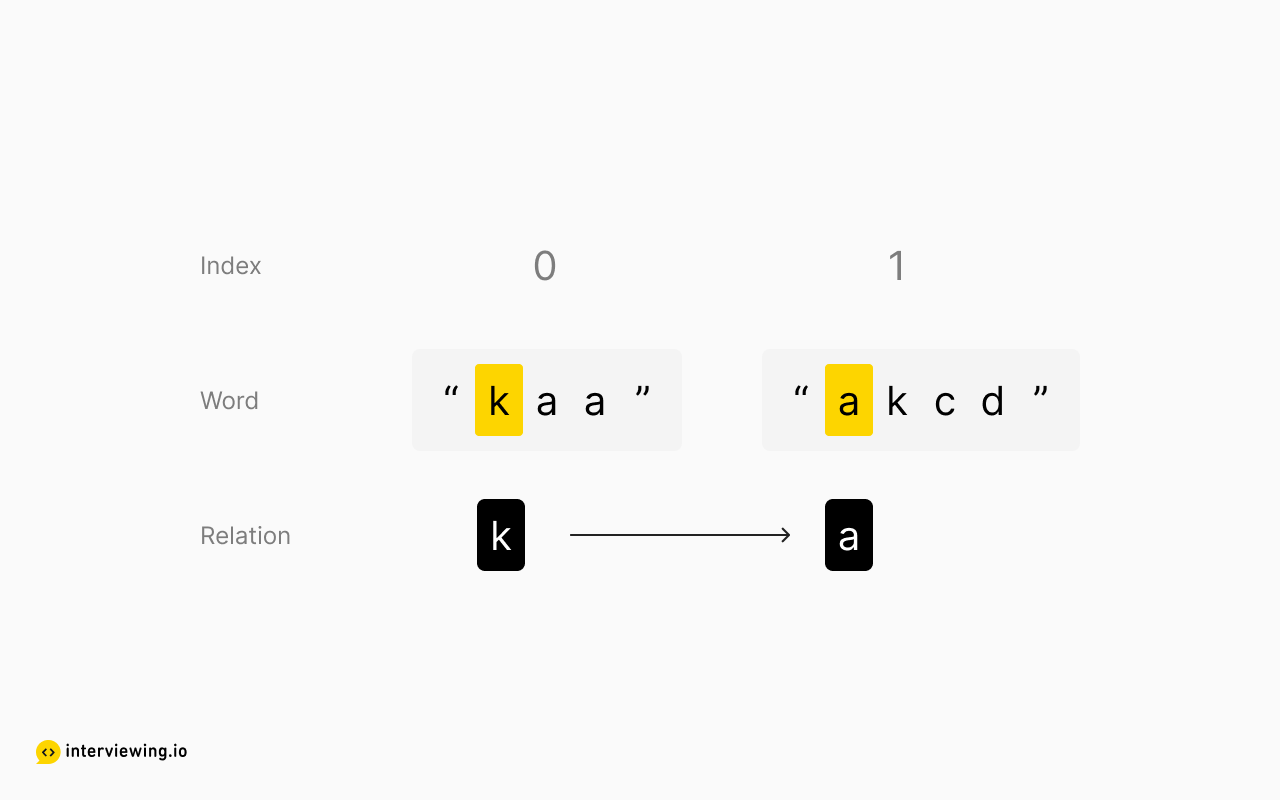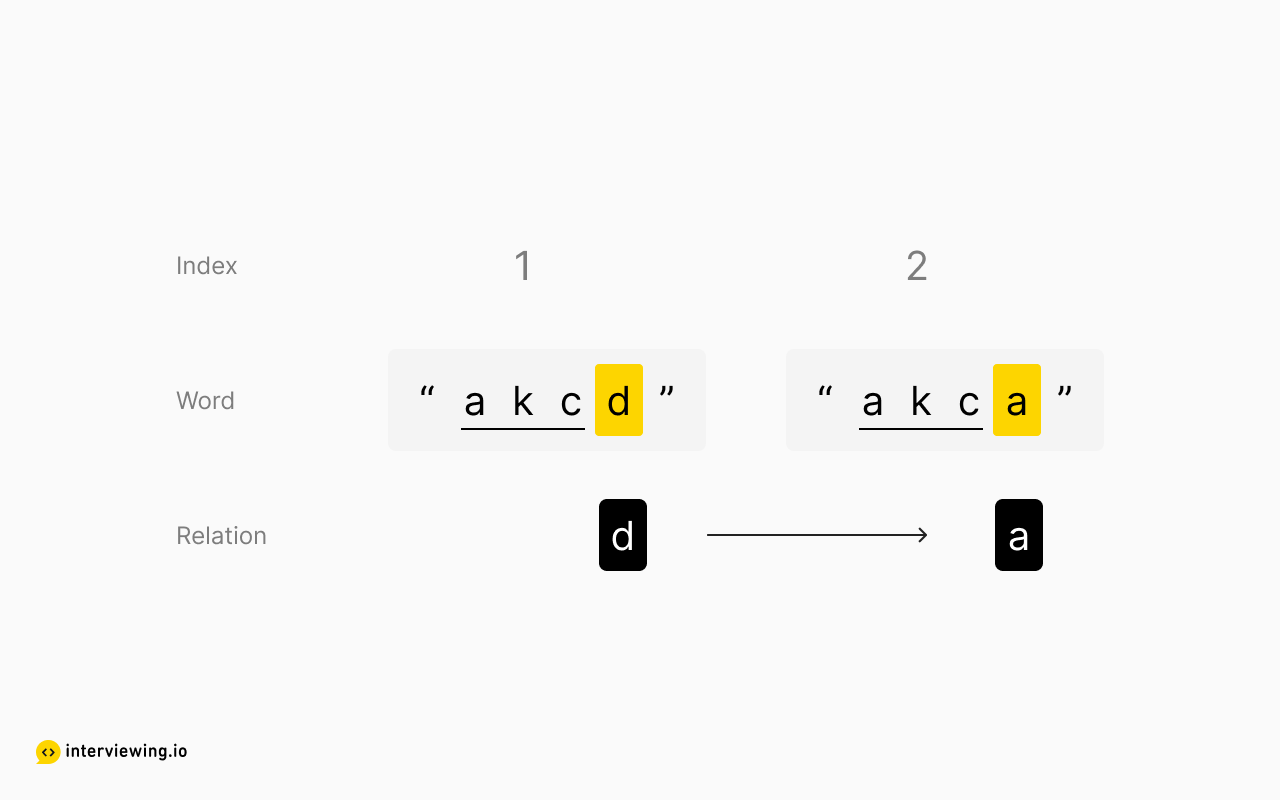Notice that we don't necessarily know exactly how these two groups relate to one another, but to build a graph we don't need absolute relationships.

What else can we deduce? Well, lexicographic order doesn't stop at the first letter - if the first letters are the same, then order is determined by the second letters. If those are the same, then the third, and so forth, until we find the first two unequal characters. So we can also infer from the second and third words: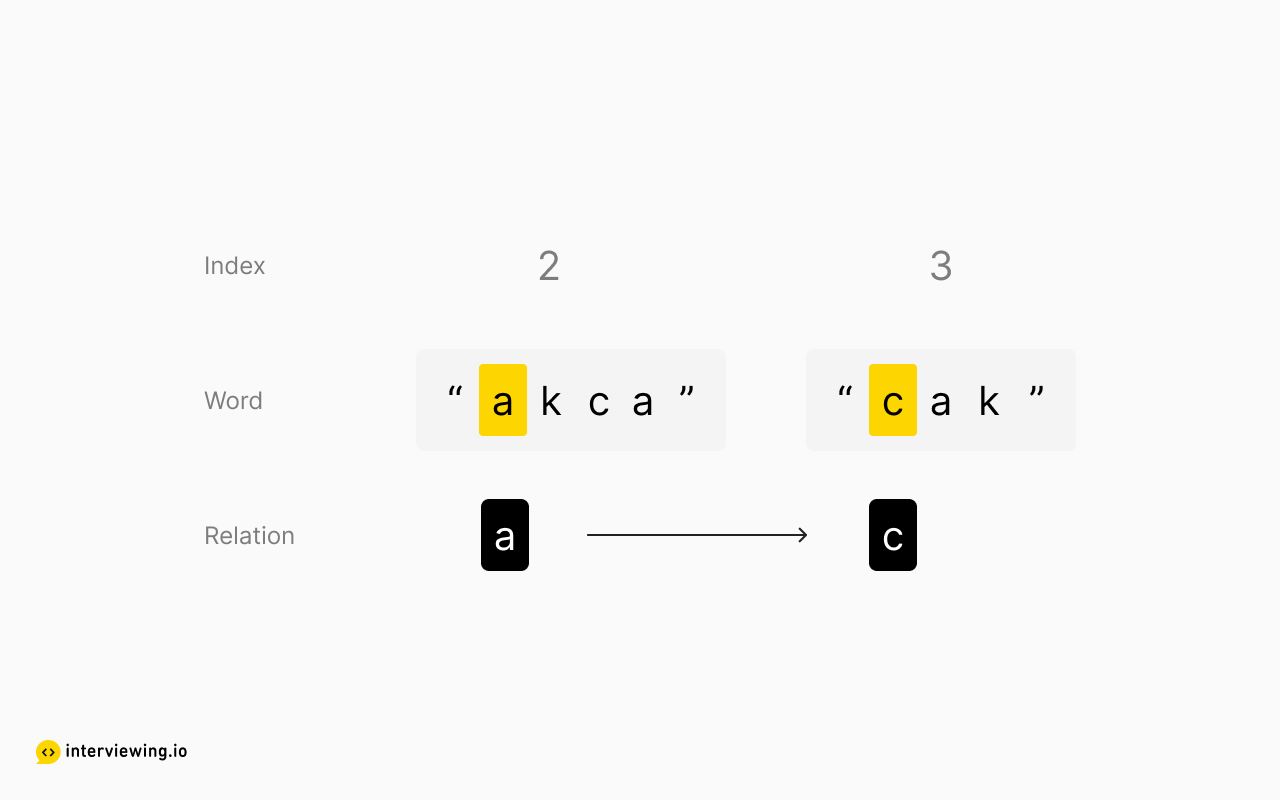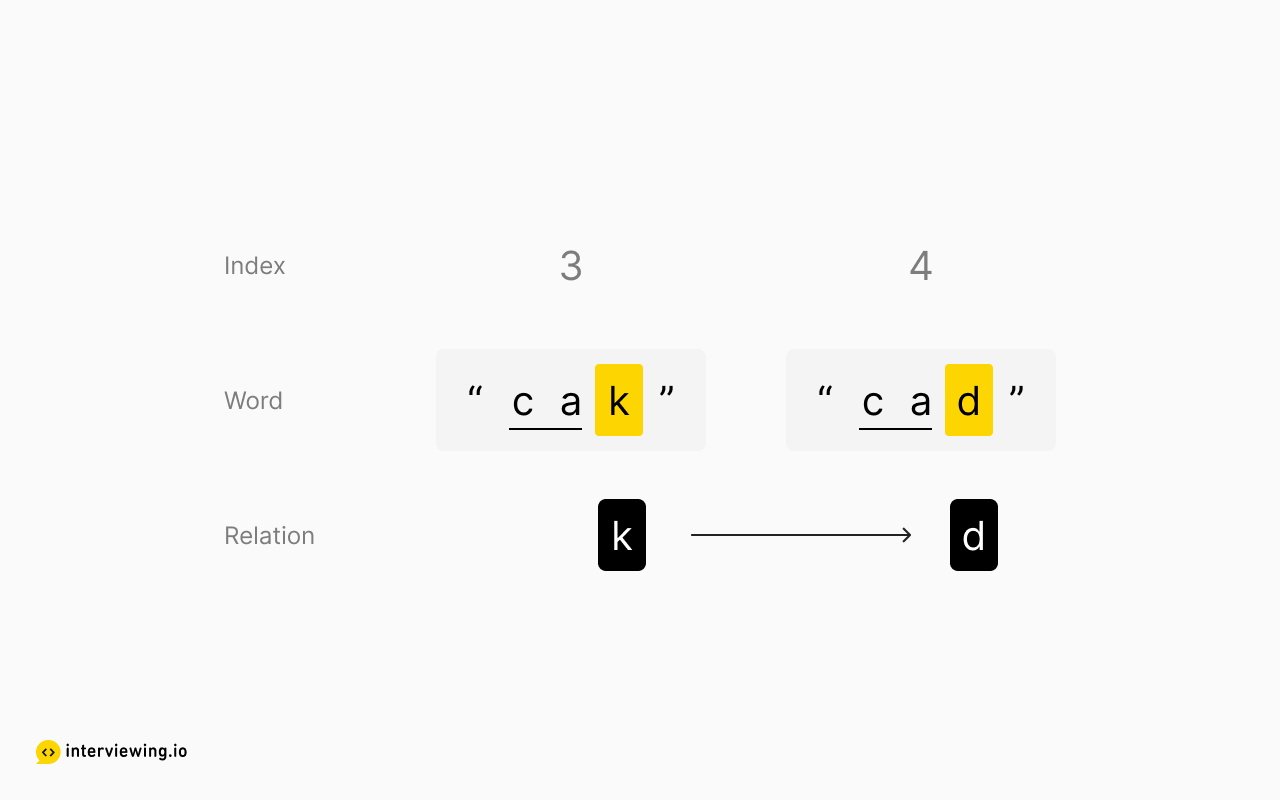Is there anything else we can determine conclusively? Nope! The order is arbitrary beyond the first different characters between two adjacent words, since the position of any subsequent letters does not impact lexicographic order. Here's a recap of what we were able to deduce for certain:

``````k -> a
d -> a
a -> c
k -> d``````

Step 2: Build the graph

Now that we have these relations, how can we use this information to build a data structure that will allow us to detemine a possible path through each character? It may be tempting to try to build the final ordered list directly, but we quickly notice that determining global order isn't a trivial task (especially for large inputs). For instance, since "k" comes before both "a" and "d", its not immediately obvious which should come next.

A directed graph will allow us to represent both of these possibilities, since we can show how multiple characters will come after a given character. All we need to build the graph is a list of vectors and their respective edges (the connections to any other vectors). This can be accomplished with an adjacency list: we'll use a hash map to store each vector as a key, with the corresponding value being a list of any subsequent characters.

``````adjacency_list = {
k: [a, d],
a: [c],
c: [],
d: [a]
}``````

Here's a visual representation of our graph: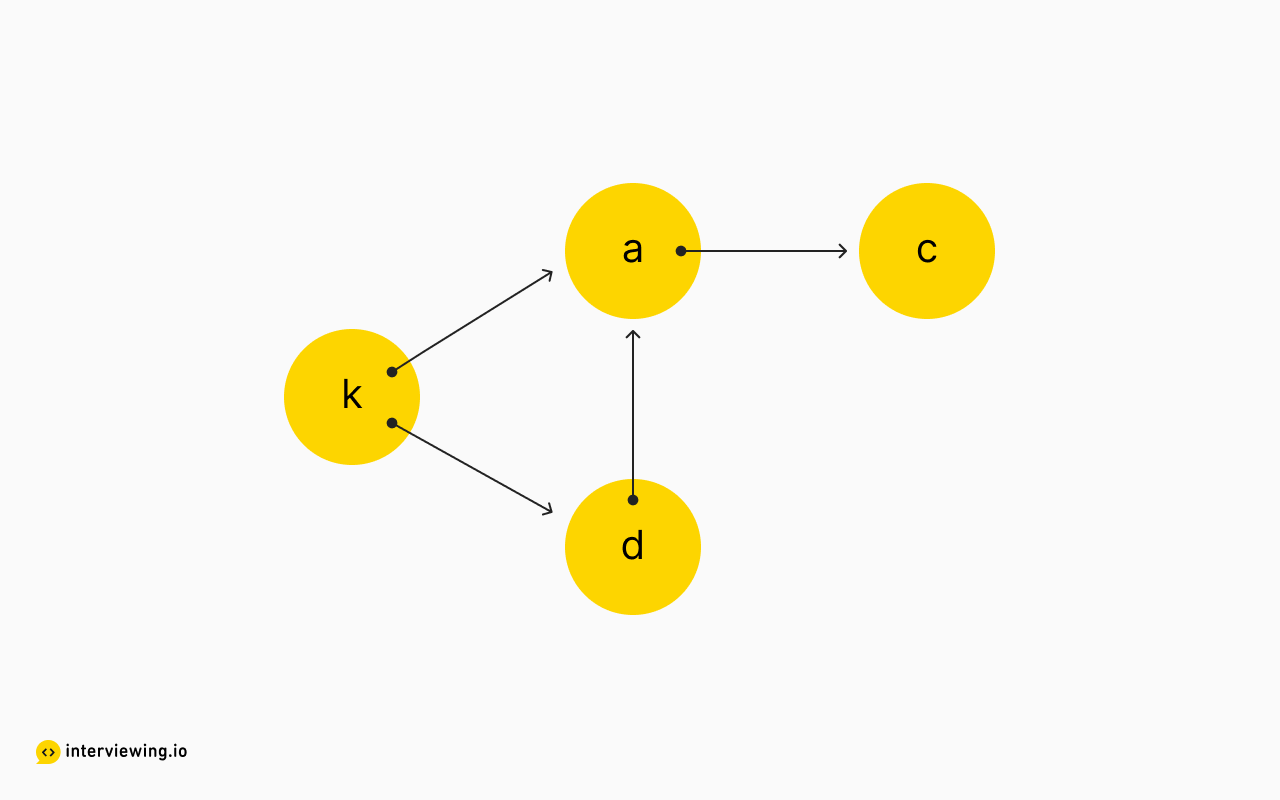Now that we have derived the relational data from the input list and represented it with our graph, we'll look at two approaches for the final task: finding a path that connects each character. One thing to keep in mind - as a constraint of the problem, we need to determine an order that includes all the characters. Therefore, if we cannot access a character within our graph - marked by the presence of a cycle - we'll return an empty string to signify an invalid input.

#### 1. Topological Sort with Breadth-First Search

Let's introduce another concept to help solve this problem: toplogical sort. Often used for problems involving dependency chains, topological sort on a directed graph offers us two very useful outcomes: cycle detection (determining if there is no valid starting point to our alphabet or if at least one character is unreachable), and, if no cycle is detected, a possible path through each vector. The word "sort" is right there in the name - this algorithm attempts to traverse the graph in a sorted order using a modified breadth-first search. Let's unpack the method.

Practically speaking, where would one begin an ordered traversal? The beginning of course! We define the beginning as any vector with no incoming edges, or, in other words, a character that does not have any characters found to precede it. Let's create another data structure to keep track of the number of incoming connections for each vector, typically called in-degrees. While building the adjacency list above, we can use another hash map to count the number of times each character appears as an edge.

``````in_degree = {
k: 0,
a: 2,
c: 1,
d: 1
}``````

We can see that "k" is a possible starting point since there are no characters that must precede it in our alphabet. We'll implement a breadth-first approach to traverse the graph from all possible starting points. Let's use a queue to store characters that have no in-degrees, and hence have no characters that must precede it, and when vectors are pulled off the queue, we'll add them to our output list. In this case, we initialize the queue with "k" in it, as well as any other characters with zero in-degrees if there were any.

So, where to next? Well, potentially any of the vectors that "k" connects to are part of a valid path ("a" or "d"), but according to the topological sort algorithm, we can only visit vectors with zero in-degrees. Luckily, once "k" is visited, it is effectively removed from the search space, so we need to update our `in_degree` counts, since "a" and "d" lost an incoming connection.

``````in_degree = {
k: 0,
a: 1,
c: 1,
d: 0
}``````

We can imagine "k" was removed from the graph: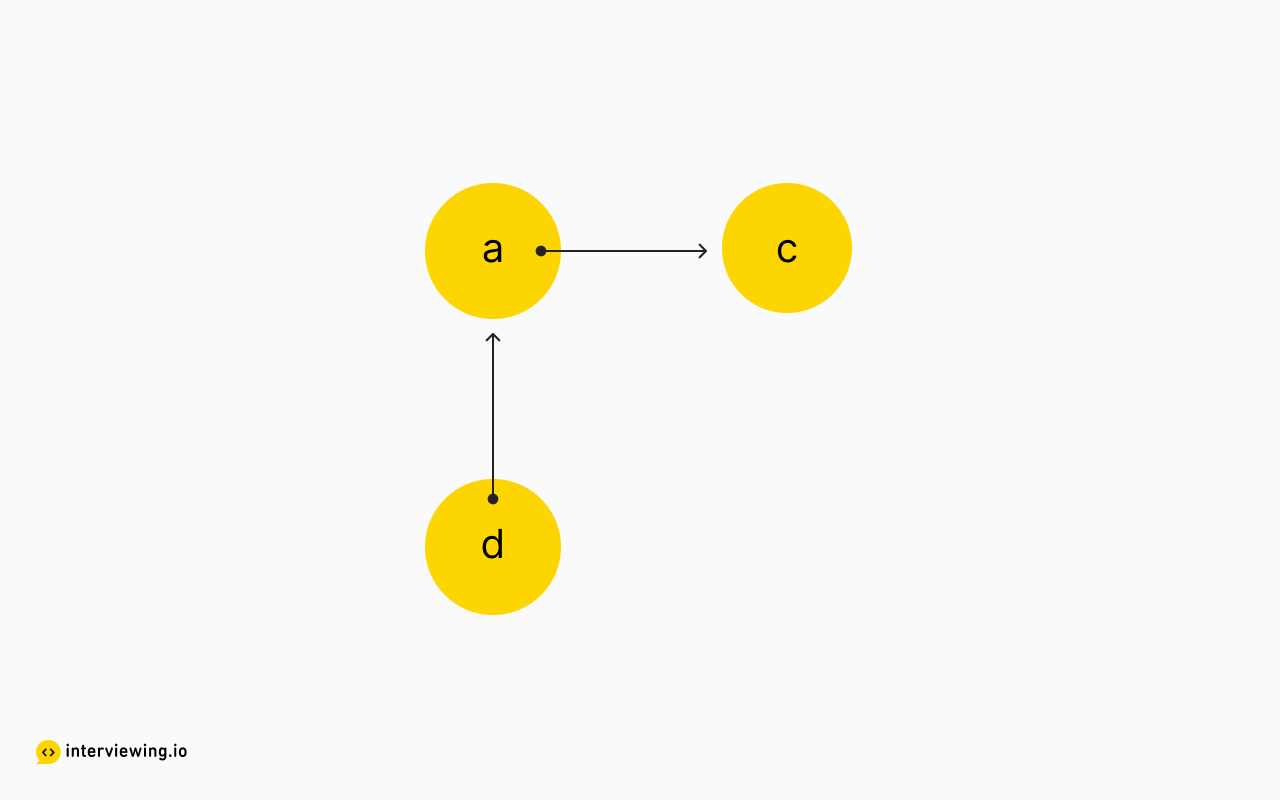With this update, we can now add "d" to the queue of vectors with zero in-degrees and continue this process until the queue is empty. And the convenience of the queue is we can store multiple vectors with zero in-degrees to be eventually processed.

This is the basic pattern of topological sort. Once the queue is empty, we check if the number of elements in our output list matches the number of vectors in our graph - if so, we have successfully traversed each vector (no cycle is detected), and we return this as a possible answer.

#### Alien Dictionary Python and JavaScript Solution: Topological Sort with Breadth-First Search

Feel free to explore the code snippets below to understand how to solve the Alien Dictionary problem in Python and JavaScript using BFS!

``````from collections import defaultdict, Counter, deque
class Solution:
def alien_alphabet(self, words: List[str]) -> str:
output = []
# 1: Populate adjacency list and in-degree list
in_degree = Counter({char: 0 for word in words for char in word})
for i in range(len(words) - 1):
word_1 = words[i]
word_2 = words[i + 1]
# Edge case: word 2 is a shorter prefix of word 1
if len(word_1) > len(word_2) and word_1.startswith(word_2):
return ''
for start_char, end_char in zip(word_1, word_2):
if start_char != end_char:
in_degree[end_char] += 1
break
# 2: Topological sort
# Populate queue with zero in-degree nodes
q = deque([char for char in in_degree if in_degree[char] == 0])
while q:
node = q.popleft()
output.append(node)
# We are removing this node, so go through its neighbors and decrement their indegrees
in_degree[neighbor] -= 1
# If at any point a vector has no indgrees left, add it to the queue
if in_degree[neighbor] == 0:
q.append(neighbor)
# Cylce detection: if we visited fewer nodes than there are vectors in our graph, there's a cycle - return an empty string
if len(output) < len(in_degree): return ''
return ''.join(output)``````

#### Time/Space Complexity Analysis

• Time Complexity: O(C), where C is the number of letters in all the words. In the worst case, building the adjacency list would require visiting every letter in every word.
• Space Complexity: O(1), since the number of possible characters is limited to ASCII, this remains constant regardless of the input size. Alternatively, we can mention that the adjacency list will use O(V+E) memory, where V is the number of vectors in the graph - unique characters from the input - and E is the number of edges in the graph - the relations we deduced between characters.

#### 2. Depth-First Search

As an alternative approach for traversing the graph in a sorted order, we can use depth-first search. This does require a modification to our graph though, since, as a recursive algorithm, depth-first traversal typically returns when a node with zero outgoing connections is reached (or the outgoing connections that do exist have already been visited).

This means we would effectively be building the output in reverse: the algorithm will first search for "starting points" - in this case, vectors with no outgoing connections - then gradually make its way back down the call stack, tracking the path in our output array. We can reverse this path and return the appropriate order, or, build the graph initially with edges reversed in our adjacency list (the method below) and avoid needing to reverse the output. And since our depth-first search is recursive, we won't need a queue anymore, but we will use a global set to track already visited vectors.

#### Alien Dictionary: Python and JavaScript Solution (Depth-First Search)

See how to solve this problem using DFS in the code snippets below:

``````class Solution:
def dfs(self, node: str, graph: dict, seen: dict, output: list):
if node in seen:
return seen[node]
seen[node] = False
for child in graph[node]:
result = self.dfs(child, graph, seen, output)
if not result:
return False
seen[node] = True
output.append(node)
return True
def alien_alphabet(self, words: List[str]) -> str:
# 1: Build graph
reverse_graph = {char: [] for word in words for char in word}
for i in range(len(words) - 1):
word_1 = words[i]
word_2 = words[i + 1]
# Edge case: word 2 is a shorter prefix of word 1
if len(word_1) > len(word_2) and word_1.startswith(word_2):
return ''
for start_char, end_char in zip(word_1, word_2):
if start_char != end_char:
if start_char != end_char:
reverse_graph[end_char].append(start_char)
break
# 2: Depth-first search
seen = {}
output = []
for node in reverse_graph:
if not self.dfs(node, reverse_graph, seen, output):
return ''
return ''.join(output)``````

#### Time/Space Complexity Analysis

• Time Complexity: `O(C)`, where C is the number of letters in all the words. In the worst case, building the adjacency list would require visiting every letter in every word.
• Space Complexity: `O(1)`, since the number of possible characters is limited to ASCII, this remains constant regardless of the input size. Alternatively, we can mention that the adjacency list will use O(V+E) memory, where V is the number of vectors in the graph - unique characters from the input - and E is the number of edges in the graph - the relations we deduced between characters. Footer

## Practice the Alien Dictionary Problem With Our AI Interviewer

Start AI Interview